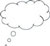# What you can learn in this unit

• How to work with your students on visualising and contextualising equations.
• Some ideas on how to use contexts to help your students to see the purpose in equations.
• How to use concept maps and mind maps to build an understanding of the mathematical concept of equations.

This unit links to the teaching requirements of the NCF (2005) and NCFTE (2009) outlined in Resource 1.

## Specialised mathematical vocabulary used in this unit

An algebraic equation is of the form p(x) = q(x):

• ‘p(x)’ and ‘q(x)’ are algebraic expressions.
• ‘p(x)’ is the expression on the left-hand side (LHS) of the equation in this example.
• ‘q(x)’ is the expression on the right-hand side (RHS) of the equation.
• ‘=’ indicates that the LHS is equivalent to the RHS.
• A solution set is ‘s’, the set of values that satisfy a given set of equations or inequalities. The sign of equality in such an equation means that one side results in the same value as the other for the solution set ‘s’.Pause for thoughtThinking about your own classroom, what are the common issues your students are struggling with when working on equations?Thinking back to your experiences as a mathematics student, what were the common issues you struggled with when working on equations?